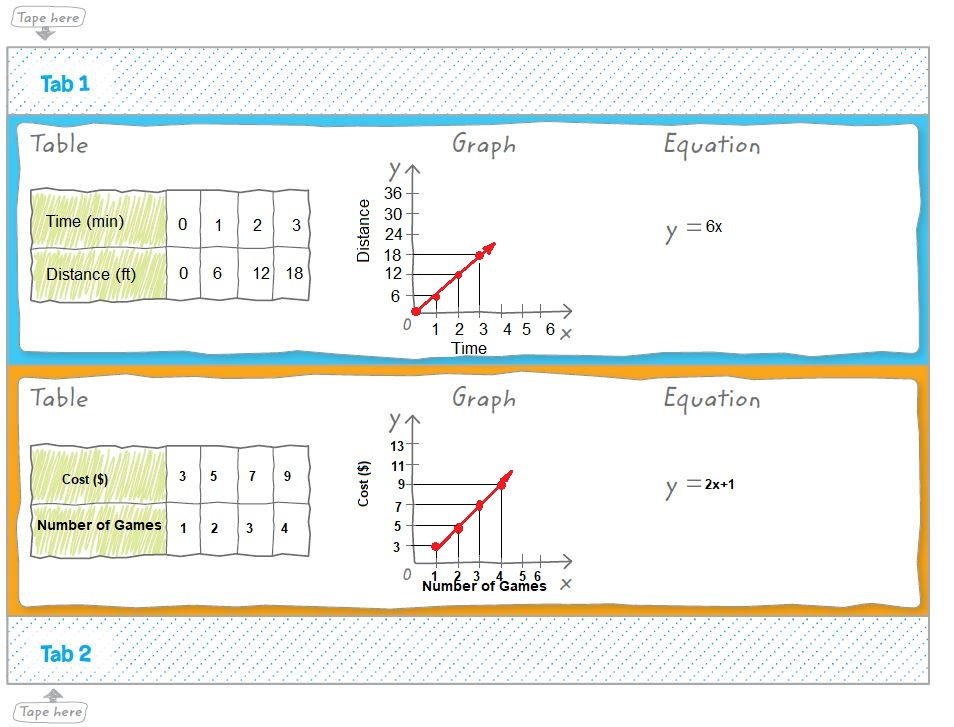Homework Explained - Math Practice 101Dear guest, you are not a registered member. As a guest, you only have read-only access to our books, tests and other practice materials.

As a registered member you can:

Registration is free and doesn't require any type of payment information. Click here to Register.
Go to page:Use your Foldable to help review the chapter.Got it?
Identify the Correct Choice Write the correct term or number to complete each sentence.

• Question 1

When a rate is simplified so that it has a (numerator, denominator) of 1 unit, it is called a unit rate.

• Question 2

If Dinah can skate $$\large\frac{1}{2}$$ lap in 15 seconds, she can skate 1 lap in (7.5, 30) seconds.

•  $$\text{s}$$
• Question 3

Slope is the ratio of (horizontal change to vertical change, vertical change to horizontal change).

• Horizontal change to vertical change
• Vertical change to horizontal change
• Question 4

When two quantities have a constant ratio, their relationship is called a (direct, linear) variation.

Yes, email page to my online tutor. (if you didn't add a tutor yet, you can add one here)